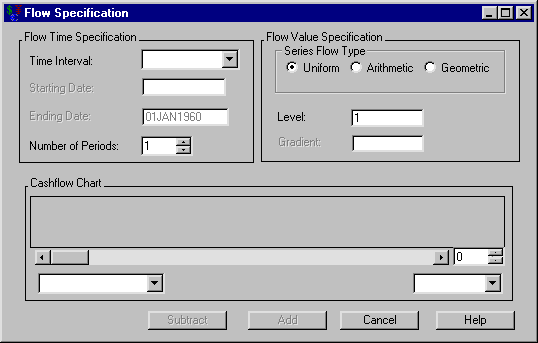Investments

Flow Specification

Figure 56.36: Flow SpecificationThe following items are displayed:

Flow Time Specification

Time Interval holds the uniform frequency of the entries.

You can set the Starting Date when you set the Time Interval. It holds the SAS date the entries will start.

You can set the Ending Date when you set the Time Interval. It holds the SAS date the entries will end.

Number of Periods holds the number of entries.

Flow Value Specification

Series Flow Type describes the movement the entries can assume:

• Uniform assumes all entries are equal.

• Arithmetic assumes the entries begin at Level and increase by the value of Gradient per entry.

• Geometric assumes the entries begin at Level and increase by a factor of Gradient per entry.

Level holds the starting amount for all flow types.

You can set the Gradient when you select either Arithmetic or Geometric series flow type. It holds the arithmetic and geometric gradients, respectively, for the Arithmetic and Geometric flow types.

When the cashflow entries are adequately defined, the Cashflow Chart fills with a graph displaying the dates and values of the entries. The box to the right of the scroll bar controls the number of entries with which to fill the graph. If the number in this box is less than the total number of entries, you can use the scroll bar to view different segments of the cashflow. The left box below the scroll bar holds the frequency.

Subtract becomes available when the collection of entries is adequately specified. Clicking Subtract then returns you to the Generic Cashflow dialog box and subtracts the entries from the current cashflow.

Add becomes available when the collection of entries is adequately specified. Clicking Add then returns you to the Generic Cashflow dialog box and adds the entries to the current cashflow.

Cancel returns you to Generic Cashflow dialog box without changing the cashflow.